# Flory-Huggins theory

(diff) ← Older revision | Latest revision (diff) | Newer revision → (diff)

The Flory-Huggins theory defines the volume of polymer system as a lattice which is divided by small subspaces (called sites) of the same volume. In the case of polymer solutions, the solvent is assumed to occupy single sites, while each polymer chain occupies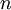$n$ sites. The repulsive forces in the system are modelled by requiring each lattice site to be occupied by only a single segment. Attractive interactions between non-bonded sites are included at the lattice neighbour level. Assuming random and ideal mixing of two polymers, i.e. mixing volume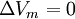$\Delta V_m = 0$ it is possible to obtain the well-known expression for the combinatorial entropy of mixing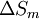$\Delta S_m$ per site of the Flory Huggins theory,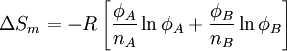$\Delta S_m = -R \left[ \frac{\phi_A}{n_A} \ln \phi_A + \frac{\phi_B}{n_B} \ln \phi_B \right]$

where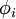$\phi_i$ is the volume fraction of the component$i$ and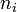$n_i$ is the number of segments in each type of polymer chain,$R$ is the molar gas constant. Applying the concept of regular solutions and assuming all pair interactions in the framework of a mean-field theory yields for the enthalpy of mixing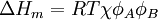$\Delta H_m = RT \chi \phi_A \phi_B$

where$T$ is the absolute temperature.

According to the preceding equations, the Flory-Huggins equation can be expressed in terms of the free-energy of mixing for a binary system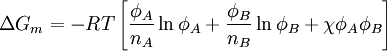$\Delta G_m = -RT \left[ \frac{\phi_A}{n_A} \ln \phi_A + \frac{\phi_B}{n_B} \ln \phi_B + \chi \phi_A \phi_B\right]$

where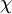$\chi$ is the Flory-Huggins binary interaction parameter, defined as: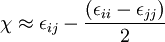$\chi \approx \epsilon_{ij} - \frac{(\epsilon_{ii}-\epsilon_{jj})}{2}$

where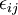$\epsilon_{ij}$ is the net energy associated with two neighbouring lattice sites of the different polymer segments for the same type or for the different types of polymer chains. Although the theory considers$\chi$ as a fixed parameter, experimental data reveal that actually$\chi$ depends on such quantities as temperature, concentration, pressure, molar mass, molar mass distribution. From the theoretical point of view it may also depend on model parameters as the coordination number of the lattice and segment length. The$\chi$ parameter is somehow similar to a second virial coefficient expressing binary interaction between molecules and, therefore, it usually shows a linear dependence of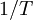$1/T$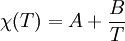$\chi(T) = A + \frac{B}{T}$$A$ and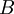$B$ are assumed to be constants but can actually depend on density, concentration, molecular weight, etc. A usual interpretation is that$A$ represents an enthalpic quantity and$B$ an entropic contribution, although both$A$ and$B$ are actually empirical parameters.

For polymers of high molecular weight (i.e.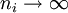$n_i \rightarrow \infty$) the entropic contribution is very small and the miscibility or immiscibility of the system mainly depends on the value of the enthalpy of mixing. In this case, miscibility can only be achieved when$\chi$ is negative. For long polymers, miscibility can only be achieved when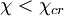$\chi < \chi_{cr}$. The$\chi$ parameter at the critical point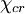$\chi_{cr}$ can be obtained from the definition of the critical point and the Flory-Huggins expression for the free-energy of mixing. The result is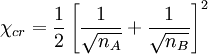$\chi_{cr} = \frac{1}{2} \left[ \frac{1}{\sqrt{n_A}} + \frac{1}{\sqrt{n_B}} \right]^2$

Therefore:

• Positive values of$\chi$ necessarily lead to incompatibility for polymers of high molecular weight.
• Mixing always take place if the$\chi$ parameter is negative.
• For a polymer solution, the critical Flory-Huggins parameter is close to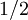$1/2$.